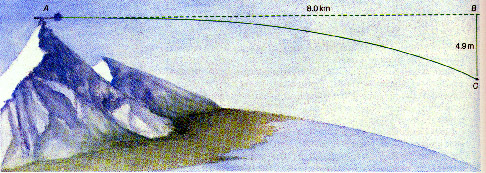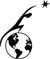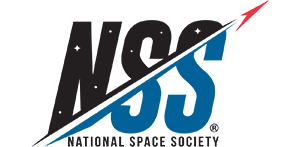# WEEK 4

## Put your space settlement into orbit...

OBJECTIVE: To determine the orbit of the space settlement CONTENT AREAS: orbital mechanics, angular motion, Newton's laws of motion In this lesson you will calculate the orbit of space settlements. The main advantage of orbital space settlements over planet based settlements is the environmental independence. In this lesson you will determine:

• the planet-moon you are going to orbit
• the angular velocity of the orbital space settlement
• the distance above the planet-moon your space settlement will orbit.

Any object with no force acting on it moves with constant velocity. This is known as Newton's First Law of motion. If a force acts on an object it would cause a change in velocity (acceleration) given by the mathematical relation: a = f/m where f is force and m is mass. This is called Newton's Second Law of motion. If you threw a ball straight forward, and there was no gravity or friction, the ball would continue on moving forward (Newton's First Law of Motion). However, on earth this does not happen because the air slows the ball due to friction and gravity pulls the ball to the center of the earth. The ball changes its vertical velocity by 9.8 meters per second per second due to the force of gravity. So if you throw a ball straight forward at first its vertical velocity is 0 meters per second. However, after one second the ball is falling towards the earth with a vertical velocity of 9.8 meters per second. After two seconds the ball has a vertical velocity of 19.6 meters per second towards earth. The faster you throw the ball the further the ball would go. What happens if you could throw the ball at a horizontal speed of 8000 meters per second with no air resistance. When the ball travels 8000 meters to point B, the earth has curved away from the ball by 4.9 meters. So even though the ball would still fall to earth continuously, because of the curvature of the earth the distance between the ball and the earth would remain constant. The ball would remain in orbit. The Space shuttle orbits the earth about 400 km above the surface of the earth. Both the space shuttle and the astronauts in it are constantly falling towards the earth. That is why the astronauts experience weightlessness.Assignments:

Author: Tugrul Sezen
[email protected]

BACK TO COURSE MAIN PAGE

 Curator: Al Globus If you find any errors on this page contact Al Globus.